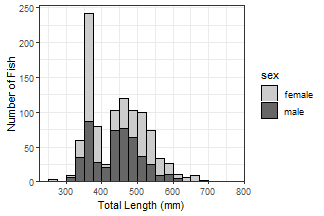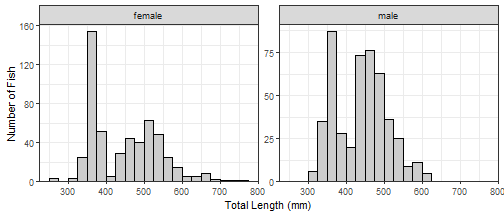Want to share your content on R-bloggers? click here if you have a blog, or here if you don't.

## Introduction

I am finally learning `ggplot2` for elegant graphics. One of the first plots that I wanted to make was a length frequency histogram. As it turns out, there are a few “tricks” to make the histogram appear as I expect most fisheries folks would want it to appear – primarily, left-inclusive (i.e., 100 would be in the 100-110 bin and not the 90-100 bin). Below are length frequency histograms that I like.

The data I use are lengths of Lake Erie Walleye (Sander vitreus) captured during October-November, 2003-2014. These data are available in my `FSAdata` package and formed ma of the examples in Chapter 12 of the Age and Growth of Fishes: Principles and Techniques book. My primary interest is in the `tl` (total length in mm), `sex`, and `loc` variables (see here for more details) and I will focus on 2010 (as an example).

## Basic Length Frequency

Making the histogram begins by identifying the data.frame to use in `data=` and the `tl` variable to use for the `x`-axis as an `aes()`thetic in `ggplot()`. The histogram is then constructed with `geom_hist()`, which I customize as follows:

• Set the width of the length bins with `binwidth=`.
• By default the bins are centered on breaks created from `binwidth=`. The bins can be changed to begin on these breaks by using `boundary=`. The value that `boundary=`, which is set to the beginning of a first break, regardless of whether that break is in the data or not. I use `boundary=0` so that bins will start on breaks that make sense relative to `binwidth=` (e.g., 0, 25, 50, 75, etc.).
• Bins are left-exclusive and right-inclusive by default, but including `closed="left"` will make the bins the desired left-inclusive and right-exclusive.
• The fill color of the bins is set with `fill=` (I prefer a slight gray).
• The outline color of the bins is set with `color=` (defaults to the same as `fill=`; I prefer a dark boundary to make the bins obvious).

The `scale_y_continuous()` and `scale_x_continuous()` are primarily used to provide labels (i.e., `name`s) for the y- and x-axes, respectively. By default, the bins of the histogram will “hover” slightly above the x-axis, which I find annoying. The `expand=` in `scale_y_continuous()` is used to expand the lower limit of the y-axis by a `mult`iple of 0 (thus, not expand the lower-limit) and expand the upper limit of the y-axis by a `mult`iple of 0.05 (thus, the upper-limit will by 5% higher than the tallest bin so that the top frame of the plot will not touch the tallest bin). Finally, `theme_bw()` gives a classic “black-and-white” feel to the plot (rather than the default plot with a gray background).

Note that the resultant plot was assigned to an object. Thus, the object name must be run to see the plot.This base object/plot can also be modified by adding (using `+`) to it as demonstrated later.

## Bins Stacked by Another Variable

It may be useful to see the distribution of categories of fish (e.g., sex) within the length frequency bins. To do this, move the `fill=` in `geom_histogram()` to an `aes()`thetic in `geom_histogram()` and set it equal to the variable that will identify the separation within each bin (e.g., `sex`). The bins will be stacked by this variable if `position="stacked"` in `geom_histogram()` (this is the default and would not need to be explicitly set below). The fill colors for each group can be set in a number of ways, but they are set manually below with `scale_fill_manual()`.Stacked histograms are difficult to interpret in my opinion. In a future post, I will show how to use empirical density functions to examine distributions among categories. For the time being, see below.

## Separated by Other Variable(s)

A strength of `ggplot2` is that it can easily make the same plot for several different levels of another variable; e.g., separate length frequency histograms by sex. The plot can be separated into different “facets” with `facet_wrap()`m which takes the variable to separate by within `vars()` as the first argument.If the faceted groups have very different sample sizes then it may be useful to use a potentially different y-axis scale for each facet by including `scales="free_y"` in `facet_wrap()`. Similarly, a potentially different scale can be used for each x-axis with `scales="free_x"` or for both axes with `scales="free"`.Plots may be faceted over multiple variables with `facet_grid()`, where the variables that identify the rows and variables for a grid of facets are included (within `vars()`) in `rows=` and `cols=`, respectively. Both scales can not be “free” with `facet_grid()` and the scale is only “free” within a row or column.## Final Thoughts

This post is likely not news to those of you that are familiar with `ggplot2`. However, I am going to try to post some examples here as I learn `ggplot2` in hopes that hit will help others. This is the first of what I hope will be more frequent posts.

Other related (non-`ggplot2`) posts are